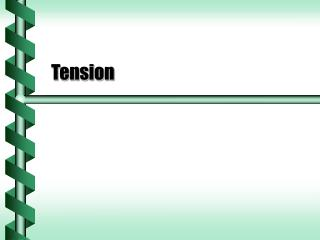DownloadDownload PresentationTension

# Tension

Télécharger la présentation## Tension

- - - - - - - - - - - - - - - - - - - - - - - - - - - E N D - - - - - - - - - - - - - - - - - - - - - - - - - - -
##### Presentation Transcript

1. Tension

2. Tension Forces • A taut rope has a force exerted on it. • If the rope is lightweight and flexible the force is uniform over the entire length. • This force is called tension and points along the rope. forces on the block forces on the rope FT FN Frope -FT Ffr m m Fg Frope = -FT by the law of reaction

3. Tension or Normal Force • Tension and normal forces are different. • A pull on an object - tension • A push from a surface - normal force • Either one or both may be present. Normal force Tension force FN FT Ffr m Fg

4. Coupled Motion v2 v1= v2 FT2 FT2 FT1 m2 m1 Ffr2 Ffr1 • Objects linked by tension move together • Same velocity and acceleration • Tension may not be the same on two ropes • FT2 = Ffr2 • FT1 = Ffr1 + FT2 = Ffr1 + Ffr2

5. m1 m1 m1 m2 m2 m2 Pulley • A pulley uses tension to transfer a force to another direction. FT forces on the rope FT forces on block 1 forces on block 2 Ffr Frope Frope Fg

6. The normal force on m1 equals the force of gravity. The force of gravity is the only force on m2. Both masses must accelerate together. m1 m2 Pulley Acceleration • Consider two masses linked by a pulley • m2 is pulled by gravity • m1 is pulled by tension • frictionless surface Ffr Frope

7. Atwood’s Machine • In an Atwood machine both masses are pulled by gravity, but the force is unequal. • The heavy weight will move downward at • (3.2 - 2.2 kg)(9.8 m/s2)/(3.2 + 2.2 kg) = 1.8 m/s2. • Using y = (1/2)at2, it will take t2 = 2(1.80 m)/(1.8 m/s2) • t = 1.4 s.

8. Mechanical Advantage • With more than one pulley the force needed to lift an object can be reduced. • The pulley is a simple machine. • The mechanical advantage to the left is 2. next